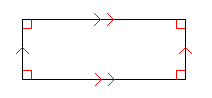# Rectangles: Area and Perimeter

A rectangle is a parallelogram with four right angles. All rectangles are also parallelograms, but not all parallelograms are rectangles.The perimeter $P$ of a rectangle is given by the formula

$P=2l+2w$ ,

where $l$ is the length and $w$ is the width.

The area $A$ of a rectangle is given by the formula

$A=lw$ .